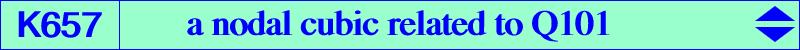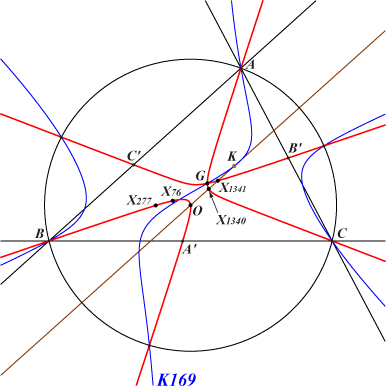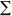b^2c^2(b^2 - c^2) x (y - z)^2 + 3a^2b^2c^2 (y - z)(z - x)(x - y) = 0X(2), X(3), X(76), X(277), X(1340), X(1341) points of K169 on the circumcircleSee Q101 for details. K657 is the isogonal transform of K055.